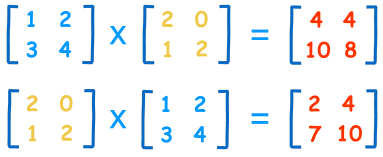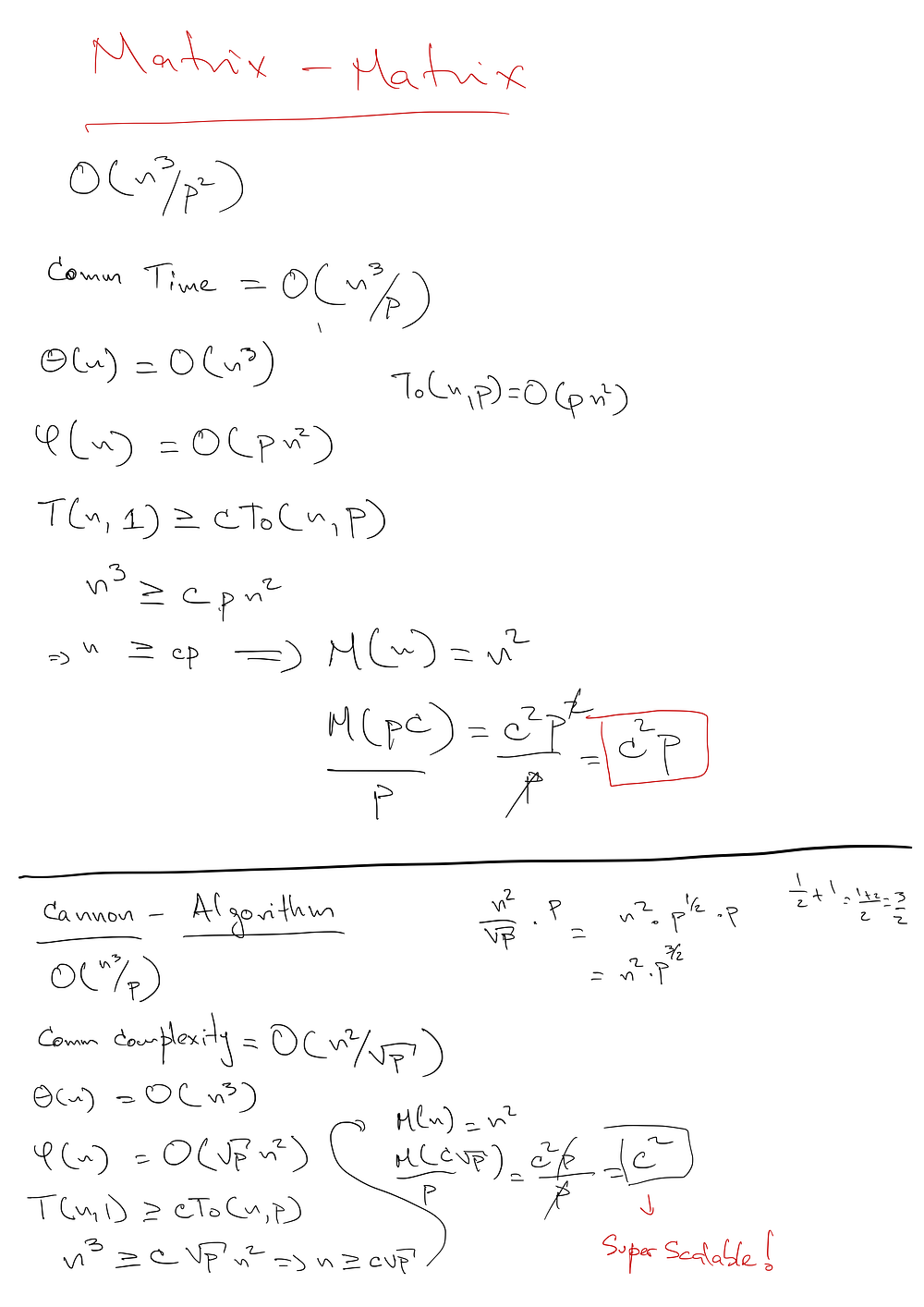Connecting...

# MPI workloads performance on MapR Data Platform Part 2 — Matrix Multiplication by Nicolas A Perez

You know how you can use MPI on top of the MapR Data Platform! Did you miss this, check it out here.

Now let's learn how to implement Matrix Multiplication in both, MPI and Apache Spark. Thanks to Nicolas A Perez, we are on our way to becoming MapR experts.In the first part of this series, we showed how we can use MPI on top of the MapR Data Platform to successfully find prime numbers within a rather large range. Also, we compare our 'Sieve of Eratosthenes' implementation in MPI and the same algorithm in Spark just to discover how they both behave while looking at some interesting implementation details.

In the second part of the series, we are going to implement 'Matrix Multiplication' in both, MPI and Apache Spark. Again, we will look at how each implementation behaves when running on MapR. However, our MPI implementation will be based on 'Cannon Algorithm' while in Spark we will use the MLlib BlockMatrix functions for multiplying matrices.

### Cannon Algorithm

There are implicit implications in parallel computing. The memory requirements tend to increase as we increase the number of processors participating in the computation. This is not trivial but proven.

The Cannon algorithm is extremely scalable. That means that by increasing the number of processors we keep the memory requirements low. The following is a scalability analysis on 'Matrix Multiplication' using 'Matrix to Matrix' multiplication against 'Block Decomposition Matrix Multiplication' used by 'Cannon Algorithm'.As we can see, in the first part ( 'Matrix-Matrix' ) we don’t get any scalability since the memory depends on 'P' — the number of processors. In the second part ( 'Cannon-Algorithm' ) the memory requirement is independent of the number of processors, more specifically, it is a constant which allows us to scale way better.

### Cannon Algorithm Implementation using MPI

Now, we are going to show the main pieces of the implementation, it’s core. Then we will link the entire source code to be reviewed for those interested in it.

First of all, let’s look at the functions we are going to use so we can grasp a better idea about the application flow.

``````1 /* set this parameter to reflect the cache line size of the particular
2    machine you're running this program */
3 #define CACHE_SIZE 1024
4
5 /* in case later we decide to use another data type */
6 #define mpitype MPI_DOUBLE
7 typedef double datatype;
8
9 void sum(int n, datatype *const *c, datatype *const *partial_c_matrix);
10
11
12 void check_input_files(char *const *argv);
13
14 /* initializes local block matrix */
15 datatype **init_partial_c_matrix(int n);
16
17 /* initializes local block matrix on a strip format */
18 datatype **init_local_c(int n, datatype **c, datatype *sc);
19
20 /* checks if a file exists */
21 int cfileexists(const char *filename);
22
23 /* re-construct a matrix from a strip format */
24 void reconstruct_matrix(int ma, int na, datatype *const *a, const datatype *sa);
25
26 /* block decomposition macros */
27 #define BLOCK_HIGH(id, p, n) (BLOCK_LOW((id)+1,p,n)-1)
28 #define BLOCK_LOW(id, p, n)  ((id)*(n)/(p))
29 #define BLOCK_SIZE(id, p, n) (BLOCK_HIGH(id,p,n)-BLOCK_LOW(id,p,n)+1)
30 #define BLOCK_OWNER(j, p, n) (((p)*((j)+1)-1)/(n))
31
32 /* Read a matrix from a file. Each processor reads only it portion of the data */
34         char *s,              /* IN - File name */
35         void ***subs,         /* OUT - 2D array */
36         void **storage,       /* OUT - Array elements */
37         MPI_Datatype dtype,   /* IN - Element type */
38         int *rows,            /* OUT - Array rows */
39         int *cols,            /* OUT - Array cols */
40         MPI_Comm grid_comm);  /* IN - Communicator */
41
42 /*
43  * Write a matrix distributed in checkerboard fashion to a file.
44  */
45 void write_checkerboard_matrix(
46         char *s,                /* IN -File name */
47         void **a,               /* IN -2D matrix */
48         MPI_Datatype dtype,     /* IN -Matrix element type */
49         int m,                  /* IN -Matrix rows */
50         int n,                  /* IN -Matrix columns */
51         MPI_Comm grid_comm);    /* IN -Communicator */
52
53 /* recursive, block-oriented, sequential matrix multiplication */
54 void my_matmul(int crow, int ccol, /* corner of C block */
55                int arow, int acol, /* corner of A block */
56                int brow, int bcol, /* corner of B block */
57                int l, int m, int n, /* block A is l*m, block B is m*n, block C is l*n */
58                int N, /* matrices are N*N */
59               datatype **a, datatype **b, datatype **c);  /* 2D matrices */``````

Notice, that reading and writing the matrices from/to file, happens in parallel and distributed. Each processor is in charge or its own block (piece) of the matrix in question.

Our application then becomes the following.

``````1 int main(int argc, char *argv[]) {
2     int p, p_sqrt;
3     int id, coord;
4     int dim, period;
5     MPI_Comm comm;
6     int ma, na, mb, nb, n;
7     datatype **a, *sa;
8     datatype **b, *sb;
9     datatype **c, *sc;
10
11     /* initialize MPI */
12     MPI_Init(&argc, &argv);
13
14     /* start couting time */
15     MPI_Barrier(MPI_COMM_WORLD);
16     double elapsed_time = -MPI_Wtime();
17
18     /* make sure the number of arguments is correct */
19     if (argc != 4) {
20         my_abort("Usage: %s fileA fileB fileC\n", argv);
21     }
22
23     /* create 2D cartesion communicator and obtain the system configurations */
24     MPI_Comm_size(MPI_COMM_WORLD, &p);
25
26     p_sqrt = (int) sqrt(p);
27
28     if (p_sqrt * p_sqrt != p) {
29         my_abort("Error: number of processors (p=%d) must be a square number", p);
30     }
31
32     dim = dim = p_sqrt;
33     period = period = 1;
34     MPI_Cart_create(MPI_COMM_WORLD, 2, dim, period, 0, &comm);
35     MPI_Comm_rank(comm, &id);
36     MPI_Cart_coords(comm, id, 2, coord);
37
38     /* checking input files are accessible */
39     check_input_files(argv);
40
41     /* read the submatrix of A managed by this process */
42     read_checkerboard_matrix(argv, (void ***) &a, (void **) &sa, mpitype, &ma, &na, comm);
43     printf("id=%d, coord[%d,%d]: read submatrix of A of dims %dx%d\n", id, coord, coord, ma, na);
44
45     if (sqrt(ma * na) != ma || sqrt(ma * na) != na) {
46         my_abort("id = %d, matrix A is not squared", id);
47     }
48
49     /* read the submatrix of B managed by this process */
50     read_checkerboard_matrix(argv, (void ***) &b, (void **) &sb, mpitype, &mb, &nb, comm);
51     printf("id=%d, coord[%d,%d]: read submatrix of B of dims %dx%d\n", id, coord, coord, mb, nb);
52
53     /* Sanity checks as necessary (such as matrix compatibility) */
54
55     if (sqrt(mb * nb) != mb || sqrt(mb * nb) != nb) {
56         my_abort("id = %d, matrix B is not squared", id);
57     }
58
59     if (ma != mb || na != nb) {
60         my_abort("id = %d, matrix A and B have different dimensions", id);
61     }
62
63     if (ma % p_sqrt != 0) {
64         my_abort("id = %d, sqrt(%d) = %d cannot divide the number of rows %d", id, p, p_sqrt, ma);
65     }
66
67     if (na % p_sqrt != 0) {
68         my_abort("id = %d, sqrt(%d) = %d cannot divide the number of columns %d", id, p, p_sqrt, na);
69     }
70
71     /* THE CANNON ALGORITHM STARTS HERE */
72
73     n = ma / p_sqrt; /* IMPORTANT: we don't have the entire matrix; only the sub */
74
75     int source, dest;
76
77     datatype **partial_c_matrix = init_partial_c_matrix(n);
78
79     MPI_Cart_shift(comm, 1, coord, &source, &dest);
80     MPI_Sendrecv_replace(sa, n * n, mpitype, dest, 0, source, 0, comm, MPI_STATUS_IGNORE);
81
82     MPI_Cart_shift(comm, 0, coord, &source, &dest);
83     MPI_Sendrecv_replace(sb, n * n, mpitype, dest, 0, source, 0, comm, MPI_STATUS_IGNORE);
84
85     reconstruct_matrix(n, n, a, sa);
86     reconstruct_matrix(n, n, b, sb);
87
88     for (int i = 0; i < p_sqrt; ++i) {
89         c = init_local_c(n, c, sc);
90
91         mat_mul(n, a, b, c);
92
93         sum(n, c, partial_c_matrix);
94
95         MPI_Cart_shift(comm, 1, 1, &source, &dest);
96         MPI_Sendrecv_replace(sa, n * n, mpitype, dest, 0, source, 0, comm, MPI_STATUS_IGNORE);
97
98         MPI_Cart_shift(comm, 0, 1, &source, &dest);
99         MPI_Sendrecv_replace(sb, n * n, mpitype, dest, 0, source, 0, comm, MPI_STATUS_IGNORE);
100
101         reconstruct_matrix(n, n, a, sa);
102         reconstruct_matrix(n, n, b, sb);
103     }
104
105     /* write the submatrix of C managed by this process *
106     write_checkerboard_matrix(argv, (void **) partial_c_matrix, mpitype, ma, mb, comm);
107
108     /* final timing */
109     elapsed_time += MPI_Wtime();
110
111     if (!id) {
112         printf("elapsed time: %lf\n", elapsed_time);
113     }
114
115     MPI_Finalize();
116
117     return 0;
118 }``````

As we can see, this is not a simple piece of code, but it presents the core elements on 'Cannon Algorithms'. The entire source code can be found in this Github Repository.

In Spark, the same can be achieved by simple constructs. The following code snippet shows this process.

``````1 def multiply(input: String)(implicit sc: SparkContext) = {
2     val mARows = sc
3       .textFile(input)
4       .zipWithIndex()
5       .map { case (line, idx) =>
6         val values = line.split(" ").map(_.toDouble)
7
8         IndexedRow(idx, Vectors.dense(values))
9       }
10       .repartition(16)
11
12     val blockMat = new IndexedRowMatrix(mARows).toBlockMatrix()
13
14     time(blockMat.multiply(blockMat).blocks.count())._2
15   }``````

As we can see it is very simplistic, but let’s see how fast it is compared to our MPI version.

### Using MPI

1000x1000 matrix multiplication

``````mpirun -np 16 multiply 1000x1000.in 1000x1000.in 1000x1000.out

elapsed time: 2.082910 // around 2 seconds``````

10000x10000 matrix multiplication

``````mpirun -np 16 multiply 10000x10000.in 10000x10000.in 10000x10000.out

elapsed time: 307200 // around 5 mins``````

### In Spark

1000x1000 matrix multiplication

``````multiply("1000x1000.txt")(sc)

res19: Long = 4118 // around 4 seconds``````

10000x10000 matrix multiplication

``````multiply("10000x10000.txt")(sc)

res0: Long = 973943 // around 16 mins``````

As we can see, the time taken by Spark increases incredibly fast, yet MPI is able to run the process with a small time penalty. At the same time, when multiplying 10000x10000 matrices, I ran out of heap space in Spark and it had to be increased to 12g per executor to run this operation.

Again, there is a tradeoff we cannot avoid. That is simplicity and reliability from Spark compared to high performance but difficult of coding and maintaining from MPI.

At the end of the day, it is all about choosing the right tool for the job and MapR Data Platform is able to run without any problems workloads build on Spark or MPI.

### Notes on MPI reading from files

In order to multiply matrices, our MPI application must read the corresponding input from files. These files are stored in the MapR-FS which supports different interfaces to interact with it such as HDFS, POSIX, and others. Our 'C' code for MPI uses regular 'POSIX' calls to read the matrices so that each process reads only a part of the matrix that we call 'blocks'. When using Spark, Spark uses, when possible, data locality which helps with speeding up the process, since each processor reads its data locally from the node it is running. In MPI, that is dependable on the Map 'RPOSIX' client.

### Conclusions

Even though MPI presents some limitations when interacting with the MapR distributed file system, the overall processing time is quite impressive when compared to what Spark offers for 'Matrix Multiplication'. Spark, on the other hand, offers constructs for doing these operations with easy while keeping the entire process reliable and fault tolerant, features lacking on MPI.​

The tradeoff between simple usage and high performance has been there for years and cannot be ignored, being aware of it helps us to decide on every situation.'

This article was written by Nicolas A Perez​ and posted originally on Medium.